# Critical Path Method (CPM)

Revised December 2011

### Introduction to Network Analysis of Projects and CPM

The Critical Path Method (CPM) is one of several related techniques for doing project planning. CPM is for projects that are made up of a number of individual "activities." If some of the activities require other activities to finish before they can start, then the project becomes a complex web of activities.

• how long your complex project will take to complete
• which activities are "critical," meaning that they have to be done on time or else the whole project will take longer
If you put in information about the cost of each activity, and how much it costs to speed up each activity, CPM can help you figure out:
• whether you should try to speed up the project, and, if so,
• what is the least costly way to speed up the project.

#### Activities

An activity is a specific task.  It gets something done.  An activity can have these properties:
• names of any other activities that have to be completed before this one can start
• a projected normal time duration
If you want to do a speedup cost analysis, you also have to know these things about each activity:
• a cost to complete
• a shorter time to complete on a crash basis
• the higher cost of completing it on a crash basis
CPM analysis starts after you have figured out all the individual activities in your project.

#### CPM Analysis Steps, By Example

This document describes the steps for doing CPM analysis for this course. The steps will be illustrated by two examples. I recommend that you work through the examples, so that you can follow the steps yourself when you do the homework.

Example 2 is especially valuable for you to work through. Excel has bugs that vary from version to version. By working through Example 2, and comparing what you get with what I got, you can find out which bugs apply to you and how to work around them when you do the assignment.

### Example 1: Activities, precedence, and times

This first example involves activities, their precedence (which activities come before other activities), and the times the activities take. The objective is to identify the critical path and figure out how much time the whole project will take.

#### Example 1 Step 1: List the activities

CPM analysis starts when you have a table showing each activity in your project. For each activity, you need to know which other activities must be done before it starts, and how long the activity takes.

Here's the example:

 Activity Description Required Predecessor Duration A Product design (None) 5 months B Market research (None) 1 C Production analysis A 2 D Product model A 3 E Sales brochure A 2 F Cost analysis C 3 G Product testing D 4 H Sales training B, E 2 I Pricing H 1 J Project report F, G, I 1

#### Example 1 Step 2: Draw the diagram

Draw by hand a network diagram of the project that shows which activities follow which other ones. This can be tricky. The analysis method we'll be using requires an "activity-on-arc" (AOA) diagram. An AOA diagram has numbered "nodes" that represent stages of project completion. You make up the nodes' numbers as you construct the diagram. You connect the nodes with arrows or "arcs" that represent the activities that are listed in the above table.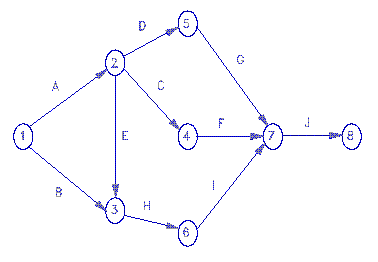Some conventions about how to draw these diagrams:

• All activities with no predecessor come off of node 1.
• All activities with no successor point to the last node, which has to have highest node number.

In this example, A and B are the two activities that have no precedessor. They are represented as arrows leading away from node 1.

J is the one activity that has no successor, in this example. It therefore points to the last node, which is node 8. If there were more than one activity with successor, all of those activities' arrows point to the highest number node.

Students sometimes make the mistake of creating a diagram with several starting or ending nodes. Don't do this.

The trickiest part for me of building the above diagram was figuring what to do with activity H. I had drawn an arrow for activity B coming off node 1 and going to mode 3. I had later drawn an arrow for activity E coming off node 2 and going to node 6. Since H requires both B and E, I had to erase my first E arrow and redraw it so it pointed to the same node 3 that B did. H then comes off of node 3 and goes to node 6.

When designing these diagrams, work in pencil.

#### Example 1 Step 3: Set up the CPM spreadsheet

There are specialized commercial programs for doing CPM analysis. Rather than purchase and learn one of those, we'll leverage the spreadsheet knowledge we already have. We will use one freeware program written for this course and made available to you through the Internet.

Start up a new blank spreadsheet. If you are viewing this document on the web, minimize your browser window and then start Excel. That way you can switch from one to the other by pressing Alt+Tab.

In a blank spreadsheet, type the word "Activities" in cell A1. In row 2, type the names of the activities, or their letters. (To make my spreadsheet screen shots fit better on these pages, I set the column widths to 4. You do not have to do this.)In row 3, type "Nodes". In row 4, type in each activity's start node -- where the tail of its arrow is. Below that, in row 5, type each activity's end node -- where the head of its arrow is. Do this carefully. Mistakes here mess up everything that follows.

To the right, in K2 and K3, type the words "Start" and "End" to label those rows.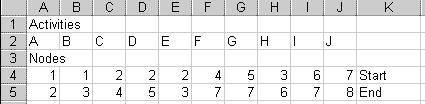In cell A6, type "Times". In row 7, type the time each activity takes. Then, select the range of cells containing the node numbersand copy it to the clipboard.

#### Example 1 Step 4: Use Pathfind to get the paths

Pathfind is a computer program that helps you find and enter into the spreadsheet all of the possible paths through your diagram along the arrows from the first node to the last. You could do this by hand, of course. This diagram shows the four possible paths in this example.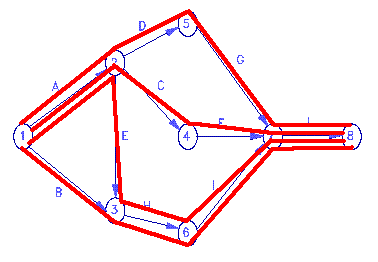The four paths are A D G J, A C F J, A E H I J, and B H I J. We'll code them in the spreadsheet with a matrix of 0's and 1's. Rather than do this all by hand, we'll get Pathfind help do it.

To use Pathfind now, go to Pathfind.

Pathfind is a Java applet that runs inside your browser. When Pathfind is loaded:

1. Click in Pathfind's upper text area.
2. Paste the range you just copied from your spreadsheet into that upper text area. (Click in the text area and press Ctrl+V or Shift+Insert.)
3. Click on Pathfind's button. Pathfind's lower text area will give you a block of numbers, all highlighted so you can copy them.
4. Copy the highlighted numbers to the clipboard for pasting later into your spreadsheet. (Ctrl+C or Ctrl+Insert copies what is highlighted.)
You can now close the Pathfind web page, if you wish.

#### Example 1 Step 5: Paste the path information into your spreadsheet

When you're done with Pathfind, go back to your spreadsheet. Move your cell selector to cell A8. Type "Paths" in that cell. Then move the cell selector to A9, as shown here: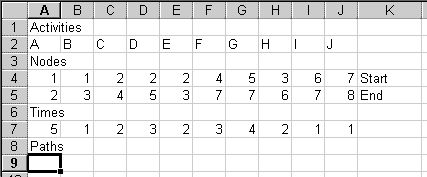Paste to that cell, to see this: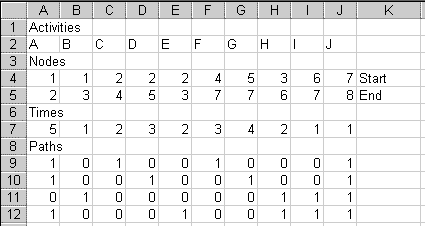The pasted cells are all 0's and 1's. Each row represents a path. The 1's indicate which activities are in that particular path. For example, row 9 (cells A9:J9) has 1's under activities A, C, F, and J. This says that this path includes activities A, C, F, and J. This corresponds to the path through the middle of the diagram that goes: 1 -A-> 2 -C-> 4 -F-> 7 -J-> 8.

The diagram above shows four paths from node 1 to node 8. Sure enough, Pathfind gives you four rows of 0's and 1's, one row for each path.

#### Example 1 Step 6: Calculate the paths' times

Move the cell selector to K9. Type =SUMPRODUCT(A9:J9,\$A\$7:\$J\$7) in that cell.
For Quattro Pro and Lotus, type @ instead of = .This formula multiplies each entry in row 9 by the corresponding entry in row 7. Because the entries in row 9 are all 0's and 1's, this has the effect of selecting the times from row 7 that go with the activities represented in row 7, and adding all those times.

When you enter the formula, the number 11 should appear in K9. That's the time it would take to complete activities A, C, F, and J. You can verify that A takes 5 months, C takes 2, F takes 3, and J takes 1, for a total of 11.

(If you are doing a CPM problem of your own, modify the formula so that the ranges cover the columns you actually have. This advice applies to all the formulas which follow.)

To fill in the other paths' times, copy cell K9, then paste it to K9:K12. The \$ signs in the formula see to it that each path's 1's are multiplied by the corresponding numbers in row 7.#### Example 1 Step 7: Identify the critical path

The critical path is the path that takes the longest. In this example, the critical path is the one in row 10, which takes 13 months. The project will therefore take 13 months, if everything is done on schedule with no delays. The time a project takes is equal to the time of its critical path.

The 1's in row 10 tell us that the critical path is 1 -A-> 2 -D-> 4 -G-> 7 -J-> 8. As managers, we must be sure that activities A, D, G, and J are done on time. If any of those activities is late, the project will be late.

Other paths are not critical because they can waste some time without slowing the project. For example, activity C, in row 9's path, can take up to two extra months and not hold up the project.

To make it easier to see what activities are in each path, go to cell A14. Type =if(A9=1,A\$2,"") there.
The letter A should appear in cell A14.

This =if(A9=1,A\$2,"") function works this way: Inside the parentheses are three expressions separated by commas. The first expression (A9=1) is something that can be either true or false. If the expression is true, the second expression (A\$2) is shown in the cell. Otherwise, the third expression ("") is shown in the cell.

In A14, the expression A9=1 is true, so the cell shows what is in A2, which is the letter "A". If A9 had not contained a 1, the A14 would have shown a blank, which is what "" means.

Copy A14 to the clipboard. Then, starting in A14, select a range of cells that goes over to column J and down four rows. The selected range should be the same size as the space that the paths' 1's and 0's take up.

Paste. You should get this: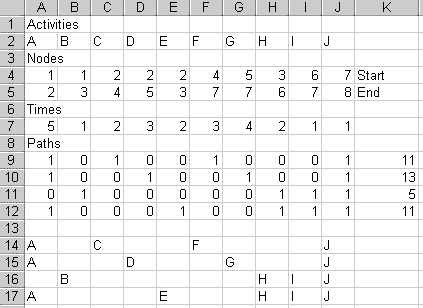Now you can see which activities are in each path. If your results do not look like the above, make sure that there is one \$ in your formula, and that it's in front of the 2 and not in front of the A.

Go to cell J13 and type "Max". Then go to cell K13. Type =MAX(K9:K12) to display the longest path time.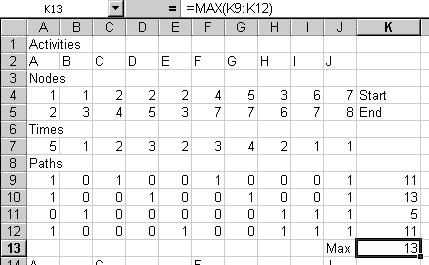Move to cell K14 and type =IF(K9=K\$13,"Critical","") there.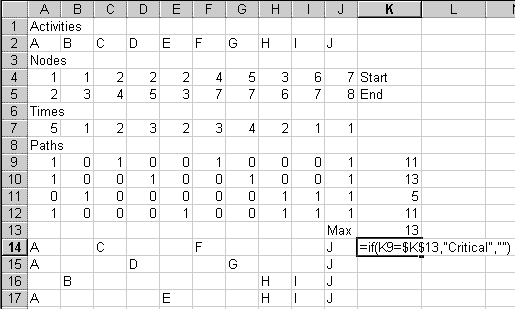This will put the word "Critical" next to a path whose time equals the maximum of all the path times. Otherwise, it will put in a blank, as it does here, because the 11 in K9 does not equal the 13 in K13.

Copy K14 to the clipboard. (It will seem strange to copy what appears to be an empty cell, but do it anyway.) Select cells K14 to K17, and paste.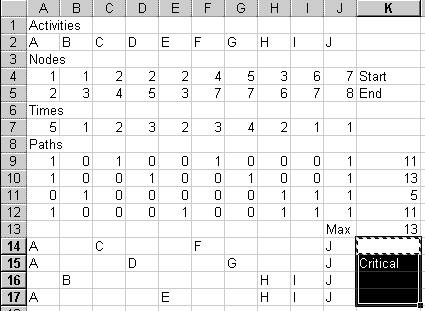You're done! You've found the time the project will take, and you have identified the critical path, which tells you which activities must be done on time to make the project finish in the least time.

Take a moment to admire your work before plunging in to Example 2.

### Example 2: Costs and Crash Costs

This second example incorporates costs and the possibility of spending money to speed up the project.  Our objective is to determine how quickly we should complete each activity, and thus the how long the project as a whole should take.  The presumption is that there is some reward for getting finished sooner.  We must decide whether the reward is worth earning, and, if so, what is the best way to earn it.

This example also shows how to use a dummy activity.  A dummy activity is an activity that you add to the original activities list.  A dummy activity takes no time, and it has no cost.  You'll learn why you sometimes need such a thing in a CPM model.

#### Example 2 Step 1: List the activities

 Activity Required Predecessor Normal Time Normal Cost Crash Time Crash Cost A (None) 3 weeks \$3000 2 weeks \$5000 B (None) 4 \$4000 2 \$6000 C (None) 5 \$5000 3 \$8000 D A 8 \$5000 6 \$6000 E A,B 3 \$3000 2 \$4000 F C 5 \$4000 3 \$8000

#### Example 2 Step 2: Draw the diagram

To start the network diagram, we notice that A, B, and C are the three activities with no predecessor. They all come off of node 1. A can go node 1 to node 2. B can go from node 1 to node 3. C also starts at node 1. D requires A, so D starts at node 2. Here's what we have so far: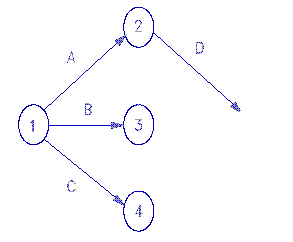Now for activity E. Activity E requires a special trick. The problem is where E should start. E requires A and B. A ends at node 2 and B ends at node 3. E is not allowed to start from both nodes 2 and 3. Activities can have only one start node and only one end node.

What do we do about E? You might consider connecting both A and B to node 2, but that would mess up Activity D. If both A and B were to run from node 1 to node 2 and D came off of node 2, that would be saying that D requires B as well as A. D is supposed to require A, not also B.

Here is the solution:The solution is to add a dummy activity that runs from node 2 to node 3, as shown in the diagram. Then start E at node 3.

If E starts at node 3, it means that E requires B and the dummy activity, the two activities that come in to node 3. The dummy activity, because it starts at node 2, requires A. This makes E require B and A. That is what we want! Meantime, D starts at node 2, so D only requires A. All the requirements are satisfied!

Dummies activities add nothing to the time or the cost. Their purpose is to allow you to represent complex relationships among activities.

#### Example 2 Step 3: Set up the CPM spreadsheet

This time we have 7 activities. That's the six lettered activities plus the one dummy activity, which we'll call Dummy.
These instructions will create the spreadsheet from scratch. Adapting the spreadsheet from example 1 is possible, but tricky, because example 2 has fewer columns and more rows than example 1.
If you start with a blank spreadsheet, move the cell selector to A1 and type "Activities". In row 2, type the activities' letters or names. Put the Dummy at the right end of row 2, for two reasons:
1. The other activities' names match will their column letters.
2. It will be easier to fill in the Solver Parameters box when we get to that stage.
In cell A3, type "Nodes".
In row 4, type in the activities' start nodes.
In row 5, type in the activities' end nodes.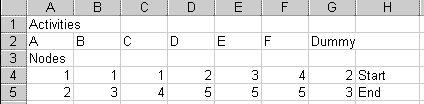Type "Times" in cell A6.
In row 7, type each activity's normal time. The dummy's time is 0.
In row 8, type each activity's crash time. The dummy's crash time is 0.

Copy row 7 and paste it into row 9. Row 9 will be the variable cells when we do the optimization later. Be sure to copy the numbers themselves from row 7 to row 9. Don't put a formula like =A7 in A9.

Label each of the Times rows by typing "Normal" in H7, "Crash" in H8, and "Actual" in H9.
(Note: When you do the homework, your number of columns will be different. These labels go in the column to the right of the last activity's information. Whatever column that is, use its letter in place of "H" in all the following instructions and formulas.)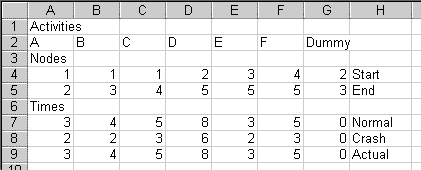When we do the optimization, we'll set a maximum time for the project and tell the spreadsheet to find the combination of numbers in row 9 that completes the project within that time for the lowest possible cost.

To do that, we need the costs. In cell A10, type "Costs".
In row 11, put each activity's normal cost. In H11, type "Normal".
In row 12, put each activity's crash cost. In H12, type "Crash".
In H13, type "Actual".
Type "0" in G13 for the Dummy's actual cost.
(When you do the homework, your column letters will differ.)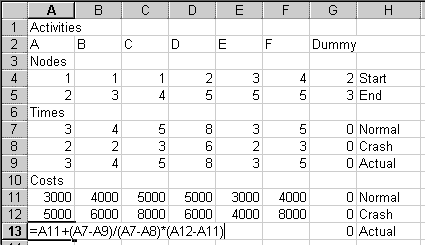The diagram above also shows a formula we'll put in A13.

Row 13 will have formulas to calculate the actual cost for each activity. Each activity's actual cost depends on how much it is sped up, or "crashed." We assume a linear relationship between speed-up and cost. So, for example, if Activity A can be shortened by 2 weeks at an added cost of \$2000, we assume that it can be shortened by 1 week for an added cost of \$1000.

The formula to implement this goes first A13. Here it is:
=A11+(A7-A9)/(A7-A8)*(A12-A11)

If you are viewing this in a web browser, you can select and copy the above formula right off of the screen. Then paste it into cell A13 of your spreadsheet.

The logic of the formula:

(A7-A9) is the difference between the normal time and the actual time we use for activity A. This difference is how much time we are saving by speeding up activity A.

(A7-A8) is the difference between the normal time and the crash time. This is the most time we could save by speeding up activity A.

(A7-A9)/(A7-A8) is how much time we are actually saving, as a fraction of how much time we could save, for activity A. In other words, it is the proportion of the possible time savings that we are actually using.

(A12-A11) is the difference between the crash cost and the normal cost for activity A. This difference is how much cost would go up if we shortened activity A's time as much as possible.
Multiplying these, to get (A7-A9)/(A7-A8)*(A12-A11), tells us additional cost we are incurring by shortening activity A's time from its normal time to the actual time we chose. This embodies the linearity assumption -- that if we go part way between the normal time and the crash time, our cost will be that same part way between the normal cost and the crash cost.
The full formula, A11+(A7-A9)/(A7-A8)*(A12-A11), adds that additional cost to A11, the cost of doing the activity in normal time. This gives us the cost of doing activity A in the amount of time in A9.

Don't paste the formula to cell G13, because that would give you a division-by-zero error. Similarly, if any of your other activities cannot be sped up (the crash time equals the normal time), put the normal cost number in the cell in row 13, not the formula.

Once that formula is in, copy cell A13 to the clipboard. Select cells A13:F13, and paste.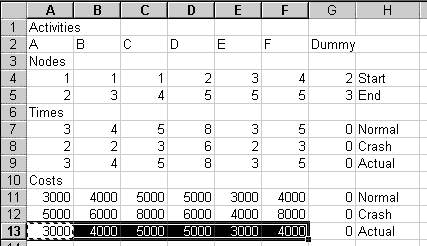Right now, it may look like the formula isn't doing much, because the Actual costs match the Normal costs. This is because the Actual times match the Normal times. Later, when we shorten the project, this will change.

Now, select the range of node numbers, being sure to include the dummy activity's node numbers.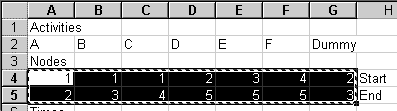Copy this range to the clipboard.

#### Example 2 Step 4: Use Pathfind to get the paths

Go to http://hspm.sph.sc.edu/Courses/J716/CPM/Pathfind.html and paste in the node numbers you just copied. Follow Pathfind's instructions for copying its reply.

#### Example 2 Step 5: Paste the path information into your spreadsheet

Go back to your spreadsheet. In cell A14, type "Paths". Then move your cell selector to A15, as shown here: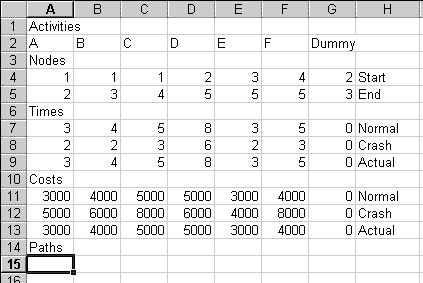Paste to that cell, to see this: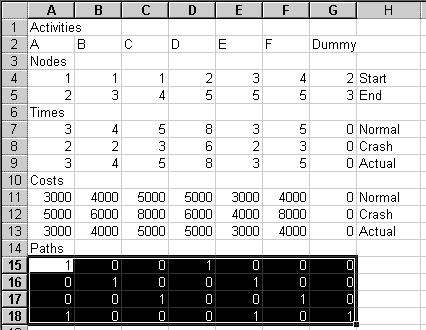As with the first example, each row represents a path. The 1's indicate which activities are in that particular path. For example, row 15 has 1's under activities A and D. This represents the path 1 -A-> 2 -D-> 5 at the top of the diagram. There are four possible paths from node 1 to node 5, so you have four rows of 0's and 1's.

#### Example 2 Step 6: Calculate the paths' times

In cell H15, put =SUMPRODUCT(A15:G15,\$A\$9:\$G\$9) . (When you do the homework, your ending column letter may differ. You want this formula to cover all of the activities.)Copy that cell and paste it to H15:H18. (When you do the homework, you will paste to a different range of cells. You will have a different number of actvities and a different number of paths.)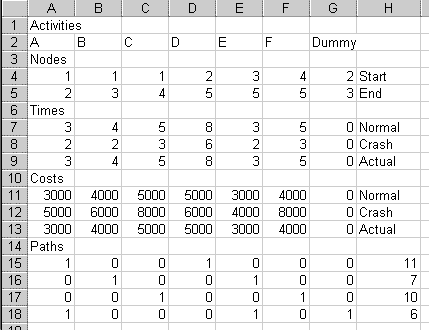We can now see how long each path takes.

#### Example 2 Step 7: Identify the critical path

The critical path is in row 15, 1 -A-> 2 -D-> 5. It's the path with the longest time.

To make it easier to see which activities are in each path, go to cell A20 and type =if(A15=1,A\$2,"") (Notice that the \$ sign is before the 2, not before the A.) This should put an "A" in A20.

Copy cell A20 to the clipboard. Select the range A20:G23. Paste.You now should have four rows showing the letters of the activities that are in each path.

Go back up to cell G19 and type "Max". Go to cell H19 and type =max(H15:H18) This shows how long the slowest path takes.

Go down to cell H20. Put in =IF(H15=H\$19,"Critical",""). In this example, "Critical" appears in cell H15, because this first path is the critical path. (In your homework, if the first path does not happen to be critical, this cell will appear blank.)

Copy cell H20. Highlight H20:H23. Paste.Sure enough, the first path with activities A and D, is the only one labelled "Critical".

#### Example 2 Step 8: Total cost formula

Go up to cell E14 and type "Total actual cost:". (In your spreadsheet, you may be able to use F14. The idea is to leave enough room so that this label does not spill over into the H column.)
In cell H14, add up the actual costs of all of the activities. The formula is =SUM(A13:G13)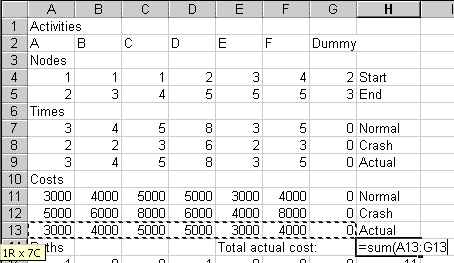When you complete the formula, you'll see that if we use all normal times for all activities, the total cost is \$24,000.

#### Example 2 Step 9: Fill in the optimizer form

Crash analysis is linear programming in disguise. To perform crash analysis, we use the Solver tool. From Excel's menu, select Tools, then Solver.

Fill in the Solver Parameters box as shown here. (For the homework, modify the formulas so they cover the rows and columns that you have.)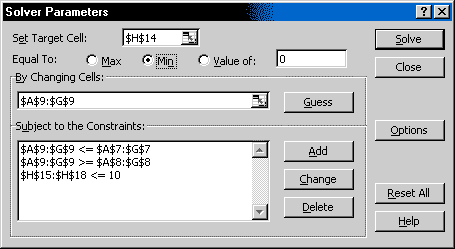• The Target Cell is H14, the total cost.
• Click on Min. Very important and easy to overlook. We want to find the least-cost way to speed up the project.
• The Changing Cells are the Actual times, in A9:G9.
• The constraints, which you add by clicking the Add button, are:
• A9:G9 <= A7:G7 All the Actual times must be less than or equal to the Normal times. We assume that we don't save any money by going slower than the Normal time. (Notice again that these formulas exclude the Dummy.)
• A9:G9 >= A8:G8 All the Actual times must be greater than or equal to the crash times. The crash times are, by definition, the fastest possible times for each activity.
• H15:H18 <= 10 The slowest path can take no more than 10 weeks. 10 is chosen because it's one less than 11, the normal completion time. Later, you can change this to 9, 8, etc., to see what happens when you try to finish the project in shorter and shorter times.

If you are using Excel 2010, choose the Simplex LP method for solution.

If you are using Excel 2000 or earlier, click on Options. Click the checkbox for assuming a linear model.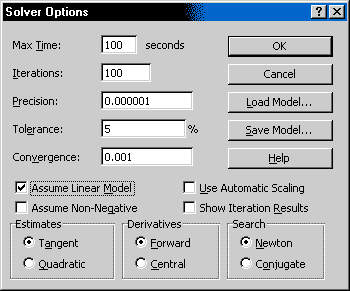Excel 2007 and 2003 do not need this, but Excel 2000 seems to. If you do not check this box, Excel 2000 may tell you at some point that there is not a feasible solution when actually there is one. Some older Excel versions, though, give wrong answers if you check this box. For older versions of Excel, my advice is to first try telling it that this is a linear model, as shown here, and see what happens when you Solve. If it won't give you a solution, uncheck the linear model checkbox and solve again.

#### Example 2 Step 10: Solve

Click on OK in the Solver Options dialog box. Click on Solve in the Solver Parameters dialog box.

If an error message appears, bring back up the Solver Parameters dialog box.

• Make sure that Min is checked. A mistake here can cause an "unbounded solution" error.
• Verify that the target cell and the changing cells are correct.
• Verify that all the constraints are correct, with greater-thans and less-thans going in the right directions. Mistakes here can cause "unbounded solution," "non-linear," and "no feasible solution" errors.
• If tried all of those, without avail, you can try the following, which are workarounds for bugs in some versions of Excel.

Bug workaround: If you get a message that there is no feasible solution, try changing the Assume Linear Model option. That is, click Solver's Options button, then check Assume Linear Model if it's unchecked, or uncheck it if it's checked.

If you still get the no-feasible-solution message, change the Solver Parameters as shown here to exclude the dummy activity from the Changing Cells and the Constraints.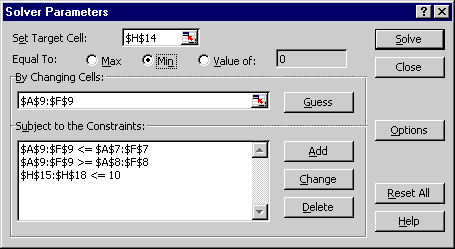The ranges for the Changing Cells and the Constraints stop at column F. Column G has the dummy activity.
Excel 2000 and later should not require this modification. Please if you have to resort to this.

Another bug workaround: If the word "Critical" does not appear everywhere that it should, Solve a second time. Just bring up Solver and click the Solve button again.

If everything is correct, a dialog box will ask you what reports you want. You can request whatever reports if you like, but I won't do anything with them here.
The result should be:To get finished in 10 weeks (H19 now has 10), we'll have to spend \$24,500 (H14 now has 24500).

As manager, you'll be busier. You now have two critical paths to worry about, 1 -A-> 2 -D-> 5, and 1 -C-> 4 -F-> 5. If any of the four activites in those paths is late, the project will take more than 10 weeks.

Let's add three more rows, to make it easier to see which activities have speeded up and at what extra cost:

Go to cell A24 and type "Crashed by how much"
Then go to A25 and put =A7-A9 there. This is the Actual time minus the Normal time.
Go to cell A26. Type in =A13-A11. This is the difference between the current Actual cost and the Normal cost.
Select A25:A26. Copy to the clipboard. Select A25:F25, and paste.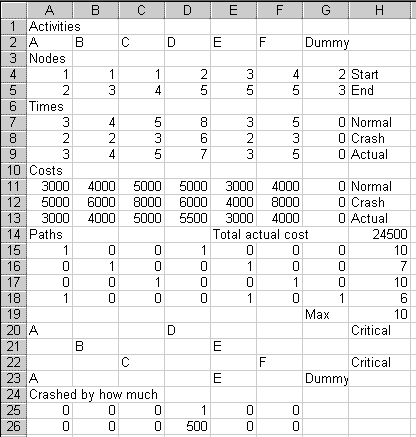We see that Activity D has been shortened by 1 week, at an extra cost of \$500.

#### Example 2 Step 11: Economic Analysis

Let's make up an economic problem to solve. Imagine that the Example 2 project is being done on a contract, with a scheduled completion time of 8 weeks. There is a \$2500 per week penalty for being late. There is also a \$1000 per week bonus for being early. Our objective is to find the best (least cost) schedule for the project. Does it pay to be on time, or are we better off paying some penalty? It is worth it to go for the bonus?

Here are our results so far. An explanation follows:

 Weeks Project cost Penalty cost Total cost 11 \$24,000 \$7,500 \$31,500 10 24,500 5,000 29,500

If we use normal times, the project takes 11 weeks, which runs over the schedule by 3 weeks. We lose \$7500 in penalties. The total project cost is \$24,000 + \$7,500 = \$31,500.

If we crash by 1 week, the project takes 10 weeks. Our penalty cost is \$5,000. Direct project cost is \$24,500, as we just saw. The total is \$29,500. This is less than \$31,500, so ten weeks is better than eleven weeks.

To try getting done in 9 weeks, go back to the Solver (step 9). Change the H15:H18 <= 10 constraint to H15:H18 <= 9. Solve. The cost rises to \$26,500. The penalty for being one week late is \$2,500. Total cost is \$26,500 + \$2,500 = \$29,000. This is less than \$29,500, so nine weeks is better than ten weeks.

 Weeks Project cost Penalty cost Total cost 11 \$24,000 \$7,500 \$31,500 10 24,500 5,000 29,500 9 26,500 2,500 29,000

Notice that cutting the second week added more to cost (second column) than cutting the first week. Cutting one week increased project cost by \$500. Cutting the second week increased project cost by \$2000, from \$24,500 to \$26,500. The law of diminishing returns is at work here.

Should we save three weeks and be on time? To try getting done in 8 weeks, go back to the Solver. Change the H15:H18 <= 9 constraint to H15:H18 <= 8. Solve. You should find that the cost is \$30,000. There is no penalty, but \$30,000 is more than \$29,500, so we lose money by being on time. We are better off being one week late and paying the penalty.

What about saving four weeks and being early? Thanks to the law of diminishing returns, we don't need to consider shorter project durations than 8 weeks. Going from 9 weeks to 8 added \$3,500 to cost. The law of diminishing returns implies that going from 8 weeks to 7 will add at least \$3,500 more to cost. That is more than the \$1000 bonus, so we know 7 weeks is a loser.

If the bonus is linear (in other words, if we get the same bonus for each additional week that we are early) then the law of diminishing returns implies that we can stop our analysis as soon as the total cost, including the bonus, starts to rise.

Here is the whole table. The bonus for being early is treated as a negative penalty.

 Weeks Project cost Penalty cost Total cost 11 \$24,000 \$7,500 \$31,500 10 24,500 5,000 29,500 9 26,500 2,500 29,000 8 30,000 0 30,000 7 Don't bother -1000 will be higher

We conclude that our optimal production schedule is 9 weeks.  It has the least total cost.

### CPM Steps Summary

CPM helps you identify a complex project's critical paths. You can find how long a project will take and which activities must be on time. If you also have information about costs and crash costs and times, CPM helps you determine how long the project should take, and which activities should be sped up ("crashed"). As we are doing it in this class, the steps are:
1. Have a list of the activities.
2. Draw the network diagram.
3. Put activity names, node numbers, times, and costs in a spreadsheet.
4. Use Pathfind to generate code for the paths.
5. Put the path information into the spreadsheet.
6. Calculate the paths' times.
7. Identify the critical paths, and the activities in each path.
8. Set up the formula to calculate the project's total cost.
9. Fill in the Tools | Solver... form.
10. Solve, and fix errors, if any.
11. For an economic analysis, change the maximum time constraint and solve again. Repeat until costs, including penalties and bonuses, start to go up.

### Assignment 12

 Activity Required Predecessor Normal Time Normal Cost Crash Time Crash Cost A (None) 4 \$2000 3 \$2600 B (None) 6 3000 3 9000 C (None) 4 8000 2 12000 D A 8 4500 5 9000 E C 6 6000 4 8000 F A 5 3000 3 6000 G C 9 6000 4 10000 H B, D, E 4 1800 3 2000 I B, D, E 6 1000 5 1200 J B, D, E 7 2000 5 3000 K F, H 8 4000 6 6000 L F, H, I 5 1500 4 2000 M G, J 6 2500 4 3000
The scheduled completion time is 24 weeks. You must pay a \$1500 penalty for every week you are late. You get a \$1000 bonus for every week you are early.

2. Find the normal completion time and the critical path.

3. Determine the schedule that minimizes your total cost for this project, including any penalty or bonus.

a. How did you decide when to stop trying shorter and shorter completion times?
b. How many weeks total should the project take?
c. What will your total cost be?
d. Which activities will be shortened from their normal times, and by how much?
e. Which activities are critical to the least cost schedule?

(You may need the two Excel Solver bug workarounds mentioned above. One is about Assume Linear Model. The other is about Solving twice.)